Search by Topic

Resources tagged with Probability distributions, expectation and variance similar to Hypothetical Shorts:

Filter by: Content type:
Age range:
Challenge level:

There are 20 results

Broad Topics > Advanced Probability and Statistics > Probability distributions, expectation and varianceA Well-stirred Sample

Age 16 to 18 Challenge Level:

Typical survey sample sizes are about 1000 people. Why is this?Statistics - Maths of Real Life

Age 14 to 18 Challenge Level:

This pilot collection of resources is designed to introduce key statistical ideas and help students to deepen their understanding.Understanding Hypotheses

Age 14 to 18Can You Find ... Random Variable Edition

Age 16 to 18 Challenge Level:

Can you create random variables satisfying certain conditions?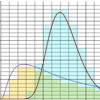Age 16 to 18 Challenge Level:

Can you work out the means of these distributions using numerical methods?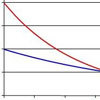Into the Exponential Distribution

Age 16 to 18 Challenge Level:

Get into the exponential distribution through an exploration of its pdf.Binomial Conditions

Age 16 to 18 Challenge Level:

When is an experiment described by the binomial distribution? Why do we need both the condition about independence and the one about constant probability?Binomial or Not?

Age 16 to 18 Challenge Level:

Are these scenarios described by the binomial distribution?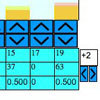Distribution Maker

Age 16 to 18 Challenge Level:

This tool allows you to create custom-specified random numbers, such as the total on three dice.Data Matching

Age 14 to 18 Challenge Level:

Use your skill and judgement to match the sets of random data.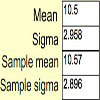Random Inequalities

Age 16 to 18 Challenge Level:

Can you build a distribution with the maximum theoretical spread?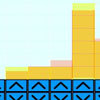Introducing Distributions

Age 14 to 16 Challenge Level:

When five dice are rolled together which do you expect to see more often, no sixes or all sixes ?Time to Evolve 2

Age 16 to 18 Challenge Level:

How is the length of time between the birth of an animal and the birth of its great great ... great grandparent distributed?Aim High

Age 16 to 18 Challenge Level:

How do you choose your planting levels to minimise the total loss at harvest time?Stats Statements

Age 16 to 18 Challenge Level:

Are these statistical statements sometimes, always or never true? Or it is impossible to say?What Is a Random Variable, Really?

Age 16 to 18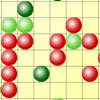Epidemic Modelling

Age 14 to 18 Challenge Level:

Use the computer to model an epidemic. Try out public health policies to control the spread of the epidemic, to minimise the number of sick days and deaths.Spinners

Age 16 to 18 Challenge Level:

How do scores on dice and factors of polynomials relate to each other?Random Squares

Age 16 to 18 Challenge Level:

What is a random pattern?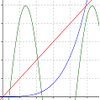Exponential Intersection

Age 16 to 18 Challenge Level:

Can the pdfs and cdfs of an exponential distribution intersect?